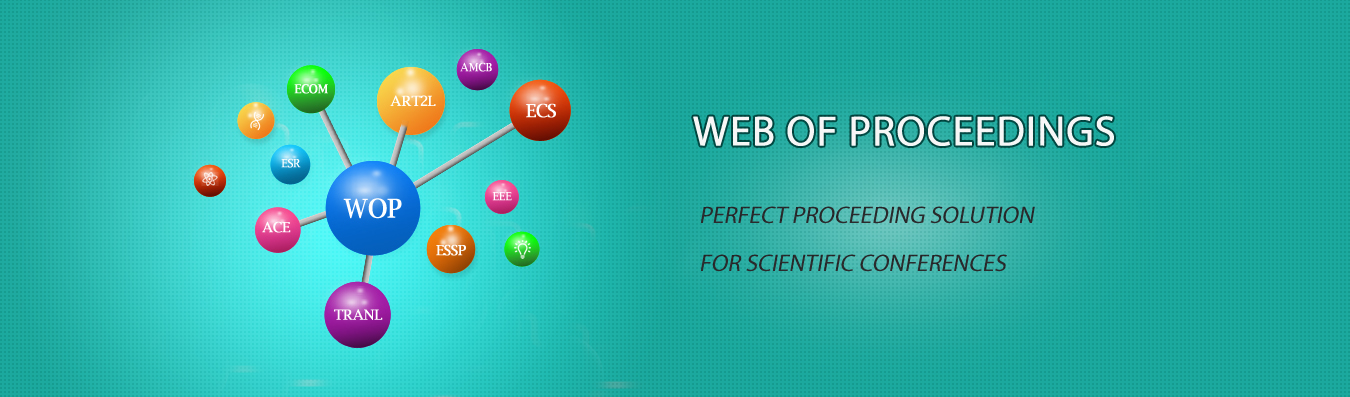The best way to conference proceedings by Francis Academic Press### The Solution for Satisfaction of Maximal Boolean Polynomial Equations Based on Genetic Algorithm

DOI: 10.25236/cape.2017.015

Shunyu Yang

Shunyu Yang

#### Abstract

In this paper, the solution for the satisfaction of Maximal Boolean Polynomial Equations based on Genetic Algorithm is proposed. The Genetic Algorithm is used to model the problem in this method, and the population of the Genetic Algorithm is taken as the solution space of the equation set and the individual is a feasible solution of the equation set. By applying the binary fitness code to the individual and selecting the appropriate individual fitness evaluation function, the more the number of the individual which make more equations with the value of 0 in the Boolean system is, the higher the corresponding fitness valueis. After the selection operation, crossover operation and mutation operation, individuals with higher fitness are retained andinherited to the next generation until the algorithm converges and eventually obtains the approximate optimal solution of the problem. Using matlab to program, the optimal solution obtained in the experimental examples is 182.

#### Keywords

Boolean polynomial equation, Optimization, Genetic Algorithm, Fitness function